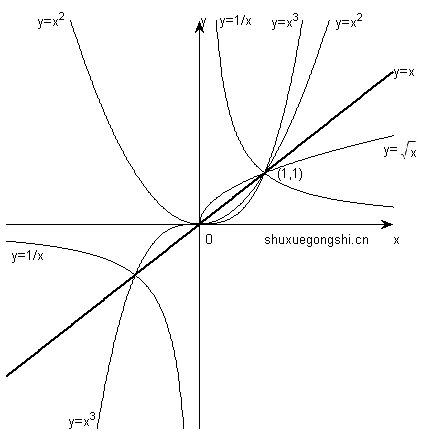## 幂函数

$y = x^{mu}$• 函数图像过(1,1)点;

• $x>0$ 时, $y=x^mu$都有定义;
所以我们常常关心的就是第一象限中各个幂函数的性质;

• 在第一象限, 当 $mu>1$ 时,x的值越大： 在y=1的上方,图像走势越接近y轴；在y=1的下方,图像走势越接近x轴(这一点在认识泰勒计算展开时，为什么要把高阶无穷小标记为 $o(x)$时非常有用 );

## 小知识：

1. 泰勒将任何可导函数f(x)都统一为了幂函数的叠加，实现了统一运算;